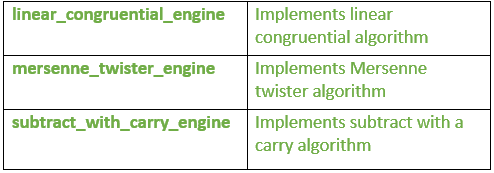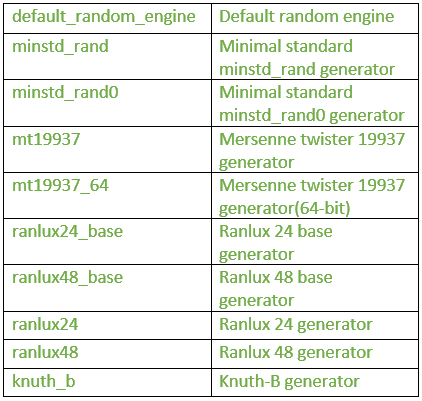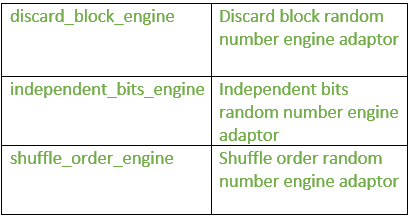# random header in C++ | Set 1(Generators)

• Difficulty Level : Medium
• Last Updated : 20 Apr, 2022

This header introduces random number generation facilities. This library allows to produce random numbers using combinations of generators and distributions.

• Generators: Objects that generate uniformly distributed numbers.
• Distributions: Objects that transform sequences of numbers generated by a generator into sequences of numbers that follow a specific random variable distribution, such as uniform, Normal or Binomial.

Generators

I. Pseudo-random number engines: They use an algorithm to generate random numbers based on an initial seed. These are:1. linear_congruential_engine: It is the simplest engine in the STL library that generates random unsigned integer numbers. It follows:

``` x = (a.x +c) mod m
Where x= current state value
a = multiplier parameter ; if m is not zero,
this parameter should be lower than m.
c = increment parameter ; if m is not zero,
this parameter should be lower than m.
m = modulus parameter ```
• operator(): It generates random number.
• min: It gives the minimum value returned by member operator().
• max: It gives the maximum value returned by member operator().

## C++

 `// C++ program to illustrate``// the use of operator(), max and min``// in linear_congruential_engine``#include ``#include ``#include ``using` `namespace` `std;` `// driver program``int` `main ()``{` `    ``// finds the time between the system clock``    ``//(present time) and clock's epoch``    ``unsigned seed = chrono::system_clock::now().time_since_epoch().count();``    ` `    ``// minstd_rand0 is a standard``    ``// linear_congruential_engine``    ``minstd_rand0 generator (seed);``    ` `    ``// generates the random number``    ``cout << generator() << ``" is a random number between "``;``    ` `    ``//use of min and max functions``    ``cout << generator.min() << ``" and "` `<< generator.max();``    ` `    ``return` `0;``}`

Output:

`211182246 is a random number between 1 and 2147483646`

2. mersenne_twister_engine: It is a random number engine based on Mersenne Twister algorithm. It produces high quality unsigned integer random numbers in the interval [0, (2^w)-1].
where ‘w’ is word size: Number of bits of each word in the state sequence.

• operator(): It generates the random number.
• min: It returns the minimum value returned by member operator(), which for mersenne_twister_engine is always zero.
• max: It returns the maximum value returned by member operator(), which for mersenne_twister_engine is 2w-1 (where w is the word size).

## C++

 `// C++ program to illustrate the use of``// operator(), min and max``// in mersenne_twister_engine``#include ``#include ``#include ``using` `namespace` `std;` `// Driver program``int` `main ()``{``    ` `    ``// finds the time between the system clock``    ``// (present time) and clock's epoch``    ``unsigned seed = chrono::system_clock::now().time_since_epoch().count();``    ` `    ``// mt19937 is a standard mersenne_twister_engine``    ``mt19937 generator (seed);``    ` `    ``// use of operator()``    ``cout << generator() << ``" is a random number between "``;``    ` `    ``// use of max and min``    ``cout << generator.min() << ``" and "` `<< generator.max();``    ` `    ``return` `0;``}`

Output:

`3348201622 is a random number between 0 and 4294967295`

3. subtract_with_carry_engine: It is a pseudo-random number generator engine that produces unsigned integer numbers.
The algorithm used is a lagged fibonacci generator, with a state sequence of r integer elements, plus one carry value.

• operator(): It generates the random number.
• max: It returns the maximum value returned by member operator(), which is (2^w)-1 for subtract_with_carry_engine , where ‘w’ is the word size.
• min: It returns the minimum value returned by member operator(), which is always zero for subtract_with_carry_engine.

## C++

 `// C++ program to illustrate the use of``// operator(), min and max``// in subtract_with_carry_engine``#include ``#include ``#include ``using` `namespace` `std;` `// Driver program``int` `main ()``{``    ` `    ``// finds the time between the system clock``    ``// (present time) and clock's epoch``    ``unsigned seed = chrono::system_clock::now().time_since_epoch().count();``    ` `    ``subtract_with_carry_engine generator (seed);``    ` `    ``// use of operator()``    ``cout << generator() << ``" is a random number between "``;``    ` `    ``// use of min and max``    ``cout << generator.min() << ``" and "` `<< generator.max();` `    ``return` `0;``}`

Output:

`8606455 is a random number between 0 and 16777215`

II. Random number generator: It is a random number generator that produces non-deterministic random numbers.

• random_device: It is the true random number generator.
• operator(): It returns a new random number.
• min: It returns the minimum value returned by member operator(), which for random_device is always zero.
• max: It returns the maximum value returned by member operator().

## C++

 `// C++ program to illustrate the use of``// operator(), min and max``// in random_device``#include ``#include ``using` `namespace` `std;` `//Driver program``int` `main ()``{``    ``random_device example;``    ` `    ``cout << ``"default random_device characteristics:"` `<< endl;``    ` `    ``// use of min``    ``cout << ``"minimum: "` `<< example.min() << endl;``    ` `    ``// use of max``    ``cout << ``"maximum: "` `<< example.max() << endl;``    ` `    ``// use of entropy``    ``cout << ``"entropy: "` `<< example.entropy() << endl;``    ` `    ``// use of operator()``    ``cout << ``"a random number: "` `<< example() << endl;``    ` `    ``return` `0;``}`

Output:

```default random_device characteristics:
minimum: 0
maximum: 4294967295
entropy: 0
a random number: 3705944883```

III. Pseudo-random number engines (instantiations): These are the particular instantiations of generator engines and adaptors:1. default_random_engine: This is a random number engine class that generates pseudo-random numbers.

• min: It returns the minimum value given by operator().
• max: It returns the maximum value given by operator().
• operator(): It returns a new random number.
The function changes the internal state by one, which modifies the state value according to the given algorithm:
``` x= (a.x + c)mod m
Where x= current state value
a and c = respective class template parameters
m = class template parameter ```

## C++

 `// C++ program to illustrate the use of ``// operator(), min and max``// in default_random_engine ``#include ``#include ``#include ``using` `namespace` `std;``  ` `// Driver program ``int` `main ()``{``      ` `    ``// finds the time between the system clock``    ``// (present time) and clock's epoch ``    ``unsigned seed = chrono::system_clock::now().time_since_epoch().count();``      ` `    ``// minstd_rand0 is a standard linear_congruential_engine``    ``minstd_rand0 generator (seed); ``      ` `    ``// generates the random number``    ``cout << generator() << ``" is a random number between "``;``      ` `    ``// Use of min and max``    ``cout << generator.min() << ``" and "` `<< generator.max();``      ` `    ``return` `0;``}`

Output:

`201066682 is a random number between 1 and 2147483646`

2. minstd_rand: It generates pseudo random numbers; it is similar to linear congruential generator

• operator(): It returns a new random number. The function changes the internal state by one, which modifies the state value according to the following algorithm:
```x = (a.x + c) mod m
where x= current state value
a ,c and m=class template parameter```
• min: It returns the minimum value given by member operator().
• max: It returns the maximum value given by member operator(), which for linear_congruential_engine is (modulus-1).

## C++

 `// C++ program to illustrate``// the use of operator(), max and min``// in minstd_rand``#include ``#include ``#include ``using` `namespace` `std;``  ` `//Driver program``int` `main ()``{``      ` `   ``// finds the time between the system clock``  ``//(present time) and clock's epoch   ``  ``unsigned seed = chrono::system_clock::now().time_since_epoch().count();``    ` `  ``// minstd_rand0 is a standard``  ``//linear_congruential_engine``  ``minstd_rand0 generator (seed);``    ` `  ``// use of operator()``  ``cout << generator() << ``" is a random number between "``;``    ` `  ``//use of max and min``  ``cout << generator.min() << ``" and "` `<< generator.max();``  ` `  ``return` `0;``}`

Output:

`489592737 is a random number between 1 and 2147483646`

3. mt19937: It is Mersenne Twister 19937 generator.It is a pseudo-random generator of 32-bit numbers with a state size of 19937 bits.

• operator(): It generates a random number. The function changes the internal state by one using a transition algorithm that produces a twist on the selected element.
• max: It returns the maximum value given by operator().
• min: It returns the minimum value given by operator().

## C++

 `// C++ program to illustrate the ``// use of operator(),min and max ``// in mt19937``#include ``#include ``#include ``using` `namespace` `std;``  ` `// Driver program``int` `main ()``{``      ` `  ``// finds the time between the system clock``  ``//(present time) and clock's epoch     ``  ``unsigned seed = chrono::system_clock::now().time_since_epoch().count();``    ` `  ``// mt19937 is a standard ``  ``//mersenne_twister_engine``  ``mt19937 generator (seed);  ``  ` `  ``//use of operator()``  ``cout << generator() << ``" is a random number between "``;``    ` `  ``//use of max and min``  ``cout << generator.min() << ``" and "` `<< generator.max();``  ` `  ``return` `0;``}`

Output:

`1445431990 is a random number between 0 and 4294967295`

4. ranlux24_base: It is Ranlux 24 base generator. It’s a subtract-with-carry pseudo-random generator of 24-bit numbers, generally used as the base engine for the ranlux24 generator.

• operator(): It returns a new random number.
The function changes the internal state by calling its transition algorithm which applies a subtract-with-carry operation on the element.
• max: It returns the maximum value given by operator().
• min: It returns the minimum value given by operator().

## C++

 `// C++ program to illustrate ``// the use of operator(),min and max``// in ranlux24_base``#include ``#include ``#include ``using` `namespace` `std;``  ` `//Driver program``int` `main ()``{``      ` `  ``// finds the time between the system clock``  ``//(present time) and clock's epoch      ``  ``unsigned seed = chrono::system_clock::now().time_since_epoch().count();``  ``subtract_with_carry_engine generator (seed);``  ` `  ``//use of operator()``  ``cout << generator() << ``" is a random number between "``;``    ` `  ``//use of max and min``  ``cout << generator.min() << ``" and "` `<< generator.max();``  ` `  ``return` `0;``}`

Output:

`7275352 is a random number between 0 and 16777215`

Similar format is applicable for the other examples.1. discard_block_engine: It is an engine adaptor class template that adapts a pseudo-random number generator Engine type by using only ‘r’ elements of each block of ‘p’ elements from the sequence it produces, discarding the rest.
The adaptor keeps an internal count of how many elements have been produced in the current block.

The standard generators ranlux24 and ranlux48 adapt a subtract_with_carry_engine using this adaptor.

• operator(): It returns a new random number.
• max: It returns the maximum value given by operator().
• min: It returns the minimum value given by operator().

## C++

 `// C++ program to illustrate``// the use of operator(),min and max``// in the discard_block_engine``#include ``#include ``#include ``using` `namespace` `std;``  ` `//Driver program``int` `main ()``{``      ` `  ``// finds the time between the system clock``  ``//(present time) and clock's epoch   ``  ``unsigned seed = chrono::system_clock::now().time_since_epoch().count();``  ` `  ``// ranlux24 is a standard instantiation ``  ``//of discard_block_engine:``  ``ranlux24 generator (seed);``  ` `  ``//use of operator()``  ``cout << generator() << ``" is a random number between "``;``    ` `  ``//use of max and min``  ``cout << generator.min() << ``" and "` `<< generator.max();``  ` `  ``return` `0;``}`

Output:

`8132325 is a random number between 0 and 16777215`

2. independent_bits_engine: It is an engine adaptor class template that adapts a pseudo-random number generator Engine type to produce random numbers with a specific number of bits (w).

• operator(): It returns a new random number.
The engine’s transition algorithm invokes the base engines’s operator() member as many times as needed to obtain enough significant bits to construct a random value.
• max: It returns the maximum value given by operator().
• min: It returns the minimum value given by operator().

## C++

 `// C++ program to illustrate``// the use of operator(),min and max``// in independent_bits_engine``#include ``#include ``  ` `// It imports the symbol names in ``// std namespace and possibly in Global namespace.``#include ``#include ``using` `namespace` `std;``  ` `//Driver program``int` `main ()``{``      ` `  ``// finds the time between the system clock``  ``//(present time) and clock's epoch    ``  ``unsigned seed = chrono::system_clock::now().time_since_epoch().count();``  ` `  ``//use of independent_bits_engine``  ``independent_bits_engine generator (seed);``  ` `  ``//use of operator()``  ``cout << generator() << ``" is a random number between "``;``    ` `  ``//use of max and min``  ``cout << generator.min() << ``" and "` `<< generator.max();``  ` `  ``return` `0;``}`

Output:

`13551674127875514537 is a random number between 0 and 184467`

3. shuffle_order_engine: It is an engine adaptor class template that adapts a pseudo-random number generator Engine type so that the numbers are delivered in a different sequence.
The object keeps a buffer of k generated numbers internally, and when requested, returns a randomly selected number within the buffer, replacing it with a value obtained from its base engine.

• operator(): It returns a new random number.
The engine’s transition algorithm picks a value in the internal table (which is returned by the function) and replaces it with a new value obtained from its base engine.
• max: It returns the maximum value given by operator().
• min: It returns the minimum value given by operator().

## C++

 `// C++ program to illustrate``// the use of operator(),min and max``// in shuffle_order_engine``#include ``#include ``#include ``using` `namespace` `std;``  ` `int` `main ()``{``      ` `  ``// finds the time between the system clock``  ``//(present time) and clock's epoch    ``  ``unsigned seed = chrono::system_clock::now().time_since_epoch().count();``  ` `  ``// ranlux24 is a standard instantiation``  ``// of discard_block_engine:``  ``ranlux24 generator (seed);``  ` `  ``//use of operator()``  ``cout << generator() << ``" is a random number between "``;``    ` `  ``//use of max and min``  ``cout << generator.min() << ``" and "` `<< generator.max();``  ` `  ``return` `0;``}`

Output:

`9213395 is a random number between 0 and 16777215`

My Personal Notes arrow_drop_up